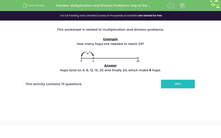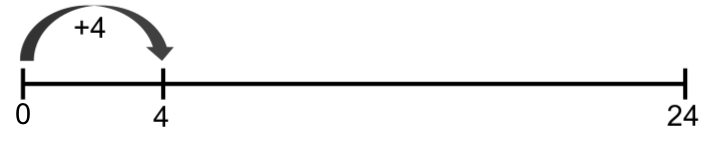# Division using a number line

In this worksheet, students resolve multiplication and division problems by using the idea of hopping along a number line to reach a target number.Key stage:  KS 2

Curriculum topic:   Number: Multiplication and Division

Curriculum subtopic:   Solve Multiplication/Division Problems

Difficulty level:#### Worksheet Overview

In this activity, we will be using number lines to solve division and multiplication problems.

We can count along a number line in equal jumps, to find out a multiplication or division fact.

This number line will help us to count up to 24 in jumps of 4.

How many hops are needed to reach 24?Jumping along the number line, we would land on 4, 8, 12, 16, 20 and finally 24, which make 6 jumps.

We could write four facts to match this counting.

4 × 6 = 24

6 × 4 = 24

24 ÷ 4 = 6

24 ÷ 6 = 4

Let's try an example together.

Example

This number line will help us to count up to 25 in jumps of 5.How many hops are needed to reach 25?

Jumping along the number line, we would land on 5, 10, 15, 20 and finally 25, which make 5 jumps.

Now it's your turn to try some questions. Good luck!

### What is EdPlace?

We're your National Curriculum aligned online education content provider helping each child succeed in English, maths and science from year 1 to GCSE. With an EdPlace account you’ll be able to track and measure progress, helping each child achieve their best. We build confidence and attainment by personalising each child’s learning at a level that suits them.

Get started